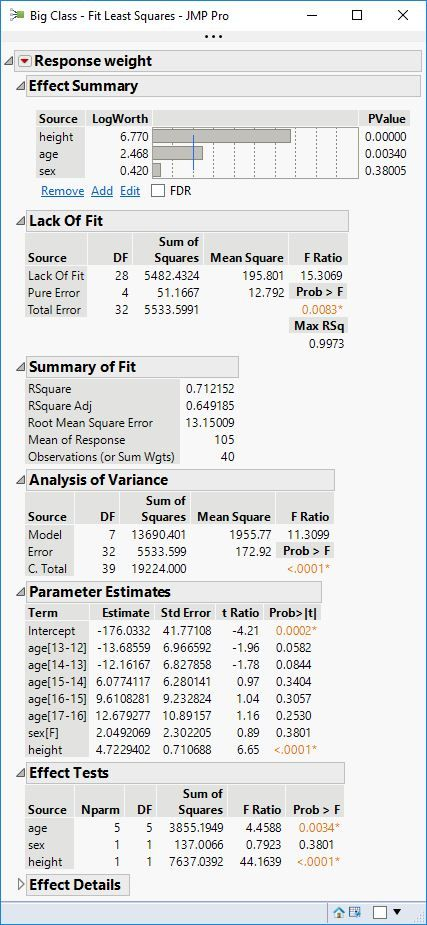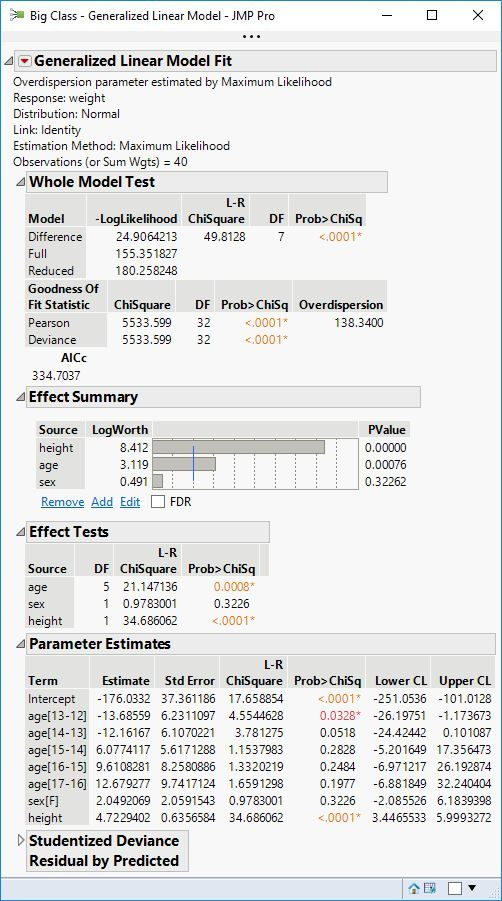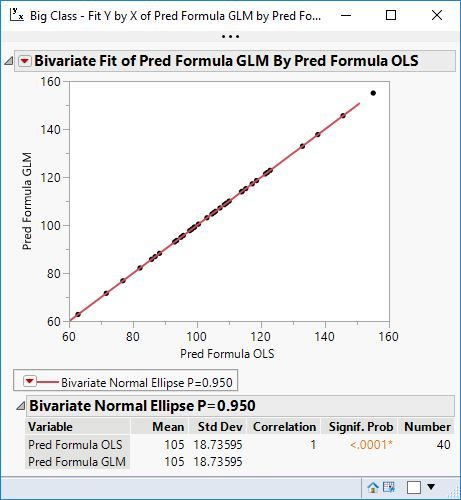Our World Statistics Day conversations have been a great reminder of how much statistics can inform our lives. Do you have an example of how statistics has made a difference in your life? Share your story with the Community!
Choose Language Hide Translation Bar
Highlighted

## ANOVA vs. GLM for ecological field experiment

I am analyzing data from an ecological field experiment, designed to test the effects of herbivore (limpet) removal and moisture addition on change in cover of a rocky intertidal seaweed. The experimental design is a randomized block, two-factor full-factorial design. I have attached a document that details the experimental design and lists the scripts and model outputs relating to three questions for the Community Discussion:

• Which modeling approach—Standard Least Squares ANOVA or GLM—is preferable for modeling results of an ecological field experiment? See Background.
• Are the scripts I used to run each type of model constructed properly? See Scripts.
• Given that the GLM (normal, identity) and ANOVA approaches both assume normal distributions, why does the GLM yield consistently smaller p values than the ANOVA? See Model output.

18 REPLIES 18
Highlighted

## Re: ANOVA vs. GLM for ecological field experiment

The GLM is a generalization of the Ordinary Least Squares Regression. They should be essentially equivalent if your case meets the assumptions of ordinary regression. Otherwise, use the GLM with a different error distribution and link function.

If you get different p-values, then there is a difference in the model specifications.

Learn it once, use it forever!
Highlighted

## Re: ANOVA vs. GLM for ecological field experiment

Thanks for your reply, Mark. Unfortunately, selecting a different error/link option isn’t possible, because the data are change values (non-integer values, including numerous observations less than zero).

I'm pasting in the scripts we constructed for ANOVA and GLM. Could you tell us from these scripts how the model specifications might differ?

ANOVA

Fit Model(

Y( :My87Ja88Mo ),

Effects( :Blk#, :Limp, :Moist, :Limp * :Moist ),

Personality( "Standard Least Squares" ),

Emphasis( "Effect Leverage" ),

Run(

:My87Ja88Mo << {Summary of Fit( 1 ), Analysis of Variance( 1 ),
Parameter Estimates( 1 ), Lack of Fit( 0 ), Expanded  Estimates( 1 ),
Scaled Estimates( 0 ), Plot Actual by Predicted( 1 ),
Plot Regression( 0 ), Plot Residual by Predicted( 1 ),
Plot Studentized Residuals( 0 ), Plot Effect Leverage( 1 ),
Plot Residual by Normal Quantiles( 0 ),
Box Cox Y Transformation( 0 )}

)

);

GLM

Fit Model(

Y( :My87Ja88Mo ),

Effects( :Blk#, :Limp, :Moist, :Limp * :Moist ),

Personality( "Generalized Linear Model" ),

GLM Distribution( "Normal" ),

Overdispersion Tests and Intervals( 0 ),

Run

);

Highlighted

## Re: ANOVA vs. GLM for ecological field experiment

The models might differ by the parameterization of the categorical factors. Please see these details.

Learn it once, use it forever!
Highlighted

## Re: ANOVA vs. GLM for ecological field experiment

Also, the estimation procedure differs. The least squares solution is a closed form that is computed directly. The GLM solution is an iterative method for maximum likelihood estimates. Here is the same model estimated first with Fit Least Squares platform:Here is the same model estimated with GLM using the normal distribution for the response with the identity link:The parameterization is the same for the categorical predicts age and sex but the estimation routines differ. The former is based on sums of squares with t and F tests, and the latter is based on likelihood with chi square ratio tests.

The two models produce identical predictions of the response:Learn it once, use it forever!
Highlighted

## Re: ANOVA vs. GLM for ecological field experiment

My thoughts:

1. There is no right way to analyze the data.  There are pros/cons to any analysis method. To over-simplify,  ANOVA basically analyzes the magnitude of the effect while GLM analyzes both the magnitude and the direction of the effect.

2. I did not evaluate your scripts as I did not have the data set.

3. I'm not sure what you mean by "Given that the GLM (normal, identity) and ANOVA approaches both assume normal distributions".  There is an assumption of the distribution of residuals, but not of the actual data set. ANOVA is fairly robust to non-normal distributions.  First, don't fall in love with the p-value statistic.  It is only an estimate.  It is a result of a comparison of mean squares.  Mean square of the treatment to the mean square error (both of which are also estimates).  If you change the comparisons or estimates, the p-value will change.

Something you might try since you are treating block as a fixed effect...saturate the model with Block, L, M, L*M, Block*L, Block*M, Block*L*M.  Use normal and Pareto plots to look for significant effects.  his removes any mean square error bias.

Highlighted

## Re: ANOVA vs. GLM for ecological field experiment

1. Thanks for the clarification of the differences between ANOVA and GLM. GLM is relatively new territory for us.

2. We are enclosing one complete column of response data from the dataset, below.

3. Both our ANOVA and GLM models use the same treatments and error terms in the Fit Model dialog. If you time to test our scripts, perhaps you can explain why the p-values differ in the outputs.

4. Thanks for the introduction to the Effects Screening module, with its normal and Pareto plot options. Not sure how to interpret significant effects, but this may not be relevant to our case.

 Block Limpets Moisture Change/mo 1 +L +M 0.5 1 +L -M 1.2 1 -L +M 0.9 1 -L -M 0.7 2 +L +M 0.5 2 +L -M 0.2 2 -L +M 1.7 2 -L -M 0.1 3 +L +M -0.2 3 +L -M 0.2 3 -L +M -0.3 3 -L -M -0.2 4 +L +M -0.2 4 +L -M 1.1 4 -L +M 0.5 4 -L -M 0.0

Highlighted

## Re: ANOVA vs. GLM for ecological field experiment

With regard to point 3, OLS is solving the normal equations directly. The results are based on sums of squares. This leads to the F test for the whole model. The normally distributed parameter estimates leads to t tests. On the other hand, the GLM is using MLE. The results are based on -2LogLikelihood. This leads to chi square tests (likelihood ratio tests).

So the estimation and tests use different methods. They are not expected to give identical p-values. Note that these values generally agree if not exactly.

Learn it once, use it forever!
Highlighted

## Re: ANOVA vs. GLM for ecological field experiment

Thank you. This is very helpful. Will GLM always yield greater
significance (lower p) than ANOVA or would this depend on the structure of
the data (e.g., deviance from the assumption of normality)?
Highlighted

## Re: ANOVA vs. GLM for ecological field experiment

No, GLM will not always produce smaller p-values than OLS for the same model.

Learn it once, use it forever!
Article Labels